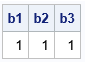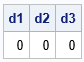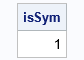It is important to be able to detect whether a numerical matrix is symmetric. Some operations in linear algebra require symmetric matrices. Sometimes, you can use special algorithms to factor a symmetric matrix. In both cases, you need to test a matrix for symmetry.

A symmetric matrix must be square. Mathematically, we say that an n x n matrix, A, is symmetric if A = AT. In terms of the elements of A, the matrix is symmetric if A[i,j] = A[j,i] for all 1 < i < j ≤ n.

### How to test a numerical matrix for symmetry

In numerical linear algebra, you should usually avoid testing floating-point numbers for equality. Instead, ask whether the difference | A[i,j] - A[j,i] | is smaller than some value, δ. But what small value should you choose for δ? Although a value such as δ = 1E-14 is suitable for many matrices, symmetry should not depend on the scaling of the matrix. In other words, the test for the matrices A and k*A should give the same results for any nonzero scalar, k.

One way to ensure that the scale of the matrix does not affect the result is to operate on a standardized matrix. You can use the elements of A to determine the scale factor. Let c = max(|A[i,j]|) for 1 ≤ i, j ≤ n. Define δ = c*sqrt(ε), where ε is machine precision. In SAS, you can use the CONSTANT('MACEPS') and CONSTANT('SQRTMACEPS') function calls to obtain the value of ε and sqrt(ε), respectively. SAS uses 2.22E-16 for machine precision, so sqrt(ε) ≈ 1.5e-8.

In summary, a good test for symmetry is to test whether all pairwise differences satisfy the equation | A[i,j] - A[j,i] | < c*sqrt(ε), where c = max(|A[i,j]|). This is equivalent to scaling A so that the largest element has unit magnitude and comparing the scaled differences with sqrt(ε).

In a matrix language such as SAS/IML, you can easily write a function that tests for symmetry, as follows:

```proc iml;   start TestSym(A); if nrow(A) ^= ncol(A) then return(0); /* A is not square */ c = max(abs(A)); sqrteps = constant('SqrtMacEps'); return( all( abs(A-A`) < c*sqrteps ) ); finish;```

### Examples of testing a matric for symmetry

With that definition, let's see whether the function works on a few example matrices. The first example is a symmetric matrix. Let's see whether the test returns 1 (true) for the matrix and for various scalings of the matrix:

```/* A is symmetric. TestSym(A) should return 1 (true). */ A = {1 2 3 -4 5, 2 3 2 3 3, 3 2 5 -1 0, -4 3 -1 4 -2, 5 3 0 -2 1 }; b1 = TestSym(A); b2 = TestSym(1E6 * A); b3 = TestSym(1E-8 * A); print b1 b2 b3;```Success. The TestSym function correctly detects that the matrix is symmetric. Various scalings of the matrix are also deemed symmetric.

What happens if we break the symmetry by making a tiny change to the (3,5) element?

```/* break the symmetry by changing an off-diagonal element */ A[3,5] = 1e-6; d1 = TestSym(A); d2 = TestSym(1E6 * A); d3 = TestSym(1E-8 * A); print d1 d2 d3;```For this matrix, the magnitude of the largest element is c=5. The perturbation to the matrix is 1e-6, which is less than c*sqrt(ε). Accordingly, the test detects that the perturbed matrix and its scalings are not symmetric. If you rerun the previous statements but use a smaller perturbation such as A[3,5] = 1e-9, the test will declare that the matrix is "numerically symmetric." Tiny differences between A[i,j] and A[j,i] are ignored, where "tiny" is relative to the magnitude of the largest element of A.

### Make a symmetric matrix

There are times when you might want to form a matrix that is symmetric from a matrix that is nearly symmetric. An easy way to do that is to use the matrix B = (A + AT)/2, as follows:

```B = (A + A`)/2; /* B is always symmetric */ isSym = TestSym(B); print isSym;```For any square matrix, A, the matrix B = (A + AT)/2 is symmetric. I call the matrix B the symmetricization of A. An off-diagonal elements B[i,j] is the average of the corresponding elements A[i,j] and A[j,i].

### Summary

This article shows how to test a matrix for symmetry in numerical linear algebra. It uses the largest value of the matrix as a scale factor to standardize the computation. This technique is used in several SAS/IML functions that need to determine whether a matrix is symmetric.

### Test for skew symmetric matrix

Here is an exercise for the motivated reader. A square matrix, M, is skew-symmetric if it satisfies the equation M = -M`. In other words, M[i,j] = -M[j,i] for all 1 < i, j ≤ n. Can you modify the TestSym function to test a matrix for a skew-symmetry to within some numerical precision? Hint: You only need to change one line!

ShareDistinguished Researcher in Computational Statistics

Rick Wicklin, PhD, is a distinguished researcher in computational statistics at SAS and is a principal developer of SAS/IML software. His areas of expertise include computational statistics, simulation, statistical graphics, and modern methods in statistical data analysis. Rick is author of the books Statistical Programming with SAS/IML Software and Simulating Data with SAS.

1.Warren F Kuhfeld on

Nice post, as always, Rick.

While you did not emphasize this point in this post, you illustrate the important point that many times in IML you can use functions, in this case ALL, to avoid writing loops that address individual matrix elements.

When I was a SAS/STAT developer, I frequently found myself faced with the question "Are these two values equal?" with the special case "Is this value equal to 0?" being the most common. I did not always choose the same method (not that I am advocating anything different in your case). I just wanted to point out that this particular section of the PROC COMPARE documentation often proved to be helpful. https://documentation.sas.com/doc/en/pgmsascdc/9.4_3.5/proc/p0bbu58eqgufwzn16zafm1hvzfw2.htm

•Thanks for writing, Warren. Two posts that are related to Warren's points are

"IF-THEN logic with matrix expressions," which discusses the ANY and ALL functions in IML
Computing absolute differences

The second post uses an expression that I use regularly: max(abs(x-y)), which yields the maximum absolute difference between two matrices or vectors, x and y.